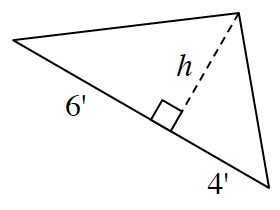### Home > CCG > Chapter 3 > Lesson 3.2.6 > Problem3-109

3-109.

The area of the triangle below is $25$ square units. Find the value of $h$. Then find the perimeter of the entire triangle. Show all work.

Use the formula for the area of a triangle to find the height.

$25=(0.5)(6+4)(h)$